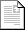Full Program »
 Final Paperpdf1.0MB

# Teaching Pid Controller Design Using Matlab

Abstract – Teaching PID Controller Design Using Matlab

Proportional Integral Derivative (PID) controllers are an industrial workhorse to achieve process control for an assortment of manufacturing physical variables such as position, speed, temperature, and pressure. This paper provides a tutorial on the design and tuning of PID controllers suitable for a course in dynamic system modeling and automatic controls for electrical or mechanical engineering students. The tutorial with its associated student project can also be used in a graduate level course in digital or optimal control theory. The paper outlines the basic theory of PID control explaining how the proportional, integral, and derivative gain setting of the controller affect the closed loop system dynamics. The impact of changing the controller parameters on the system rise time, overshoot, settling time, and steady state error is explained. The paper introduces the Ziegler-Nichols tuning rule using the reaction curve method for making an initial estimate of the PID controller parameters. A student project assignment is included in the paper to assist in reinforcing the PID concepts. The project exploits the user-friendly adaptability of the Matlab Simulink Toolbox software to move quickly from theory to practice. The simplicity of implementation encourages students to explore the trial and error nature of controller design. The trade-off between the controller performance and stability is investigated using the Matlab PIDTUNE function. This function implements an algorithm that automatically chooses the PID gains eliminating the need for trial and error. This tool provides a graphical user interface for selecting performance characteristics and produces the resulting controller gains.

Robert Barsanti